# Angle Relationships

In this lesson, you will extend your knowledge of different types of angles to learning about relationships among pairs of angles. You will also learn to write and solve equations that will allow you to find the measure of unknown angles in different figures composed of angles.

We will start out with a quick review of basic angles. Remember that angles are formed by two rays with a common endpoint (vertex). Congruent angles are angles that have the same measure. It is important to remember that when naming angles, the vertex always goes in the middle. If you need a bit more review for angle classifications, please watch the following video. Once you have seen how to name and classify angles, we will move forward to angle pairs.

In earlier years, you learned how to recognize right angles, obtuse angles, acute angles, and straight angles. We know that a right angle measures 90° and a straight angle always measures 180°.

An acute angle is an angle that measures less than 90°. How can you use the idea of a right angle to help you identify an acute angle? If the opening of an angle is smaller than the opening of a right angle, it is acute.

The same goes for an obtuse angle. This is an angle that measures more than 90 but less than 180. If the opening of an angle is larger than the opening of a right angle, but smaller than the opening of a straight angle, the angle is obtuse.

Let us look at some of the relationships that are common among angles.

Adjacent angles are pairs of angles that share a vertex and one side but do not overlap. In the examples below, angles a and b are adjacent angles.

Complementary angles are two angles whose measures have a sum of 90°. The image below shows two complementary angles. They are both acute (each measuring less than 90°) and when you add them together, they equal 90°.Supplementary angles, (also known as linear pairs), are two angles whose measures have a sum of 180°. Remember, the key word is “pair”, which means two angles. Like complementary angles, these angle pairs do not have to be adjacent. In the image below, angle a and angle b have a sum of 180°.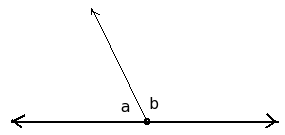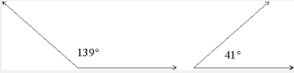Here are some basic word problems that we can solve without diagrams.

## Example 1

Angles A and B are supplementary. If m ∠ A is 32 °, what is the m ∠ B ?

Given the fact that they are supplementary, we know that the two add up to 180°. You can set up an equation to read:

B + 32 = 180 and solve for B.
-32     -32

B          = 148

The measure of angle B## Example 2

Angles C and D are complementary. If m ∠C is 22 °, what is the m ∠ D ?

Given the fact that they are complementary, we know that the two add up to 90°. You can set up an equation to read:

D + 22 = 90 and solve for D
-22   -22

D         =  68

The measure of angle D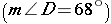You can also use diagrams to find unknown angle measures in complementary and supplementary angles.

## Example 3Angles LGM and MGH are complementary.

Create an equation to solve for x.

x + 64.9 = 90
-64.9   -64.9

x = 25.1

The measure of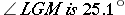.

Take a look at the video lesson to see examples of complementary and supplementary angles.

## Practice Adjacent, Complementary, and Supplementary Angles

Now, practice what you have learned about adjacent, complementary, and supplementary angles using the following diagram.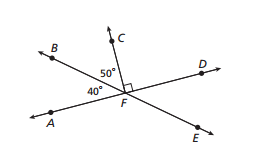1. Name the right angles.

2. Name 5 pairs of adjacent angles.

3. Name a pair of complementary angles.

4. Name an angle that is supplementary to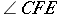.

5. Name an angle that is supplementary to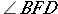.

6. Name an angle that is supplementary to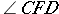.

7. Areadjacent angles?

8. Are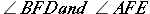adjacent angles?We have learned about angle pairs. Now, let us go a bit deeper and learn to use line and angle relationships to solve problems with figures. First of all, we know that adjacent angles have a common vertex and a common ray.

(source)